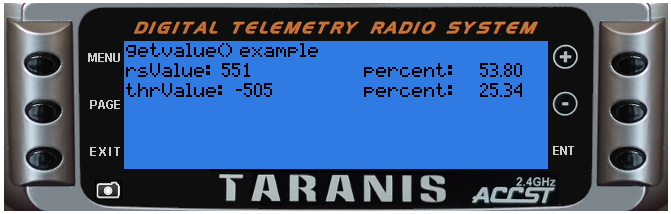## getValue(source)

Returns the value of a source.

The list of valid sources is available:

In OpenTX 2.1.x the telemetry sources no longer have a predefined name. To get a telemetry value simply use it's sensor name. For example:

• Altitude sensor has a name "Alt"
• to get the current altitude use the source "Alt"
• to get the minimum altitude use the source "Alt-", to get the maximum use "Alt+"

@status current Introduced in 2.0.0, changed in 2.1.0

#### Parameters

• `source` can be an identifier (number) (which was obtained by the getFieldInfo()) or a name (string) of the source.

#### Return value

• `value` current source value (number). Zero is returned for:

• non-existing sources
• for all telemetry source when the telemetry stream is not received
• `table` GPS position is returned in a table:

• `lat` (number) latitude, positive is North
• `lon` (number) longitude, positive is East
• `pilot-lat` (number) pilot latitude, positive is North
• `pilot-lon` (number) pilot longitude, positive is East
• `table` GPS date/time, see getDateTime()

• `table` Cells are returned in a table (except where no cells were detected in which case the returned value is 0):

• table has one item for each detected cell:
• key (number) cell number (1 to number of cells)
• value (number) current cell voltage
##### Notice

Getting a value by its numerical identifier is faster then by its name.

### Examples

general/getValue-example

``````local function run(e)
--
-- NOTE: analog values (e.g. sticks and sliders) typically range from -1024 to +1024
--       divide by 10.24 to scale into -100% thru +100%
--       or add 1024 and divide by 20.48 to scale into 0% thru 100%
--
local rsValue = getValue('rs')
local thrValue = getValue('thr')
lcd.clear()
lcd.drawText(1, 1, "getvalue() example",0)
lcd.drawText(1, 11, "rsValue: ", 0)
lcd.drawText(lcd.getLastPos() + 2, 11, rsValue, 0)
lcd.drawText(120, 11, "percent: ", 0)
lcd.drawNumber(lcd.getLastPos() + 32, 11, rsValue / 10.24, PREC2)
lcd.drawText(1, 21, "thrValue: ", 0)
lcd.drawText(lcd.getLastPos() + 2, 21, thrValue, 0)
lcd.drawText(120, 21, "percent: ", 0)
lcd.drawNumber(lcd.getLastPos() + 32, 21, (thrValue + 1024) / 20.48, PREC2)
end

return{run=run}
``````